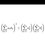# Symbol of Natural numbers

I have been commonly using $\mathbb {N}$ and $\mathbb {Z}^{+}$ to denote natural numbers. Recently I have come across on some questions the symbol $\mathbb {I}$. I have checked various websites and have not found anything to do with the I symbol. Is it a proper symbol? I will be glad to know. Probably, it is used elsewhere in the world and I am unaware.Note by Joel Tan
6 years, 2 months ago

This discussion board is a place to discuss our Daily Challenges and the math and science related to those challenges. Explanations are more than just a solution — they should explain the steps and thinking strategies that you used to obtain the solution. Comments should further the discussion of math and science.

When posting on Brilliant:

• Use the emojis to react to an explanation, whether you're congratulating a job well done , or just really confused .
• Ask specific questions about the challenge or the steps in somebody's explanation. Well-posed questions can add a lot to the discussion, but posting "I don't understand!" doesn't help anyone.
• Try to contribute something new to the discussion, whether it is an extension, generalization or other idea related to the challenge.

MarkdownAppears as
*italics* or _italics_ italics
**bold** or __bold__ bold
- bulleted- list
• bulleted
• list
1. numbered2. list
1. numbered
2. list
Note: you must add a full line of space before and after lists for them to show up correctly
paragraph 1paragraph 2

paragraph 1

paragraph 2

[example link](https://brilliant.org)example link
> This is a quote
This is a quote
    # I indented these lines
# 4 spaces, and now they show
# up as a code block.

print "hello world"
# I indented these lines
# 4 spaces, and now they show
# up as a code block.

print "hello world"
MathAppears as
Remember to wrap math in $$ ... $$ or $ ... $ to ensure proper formatting.
2 \times 3 $2 \times 3$
2^{34} $2^{34}$
a_{i-1} $a_{i-1}$
\frac{2}{3} $\frac{2}{3}$
\sqrt{2} $\sqrt{2}$
\sum_{i=1}^3 $\sum_{i=1}^3$
\sin \theta $\sin \theta$
\boxed{123} $\boxed{123}$

Sort by:

Nope, not as far as I know. I try to refrain from using $\mathbb{N}$ unless it looks super ugly otherwise, to avoid any confusion. I take $\mathbb{N} = \mathbb{Z}^{+} \cup \lbrace 0 \rbrace$ as per the Peano axioms.

- 6 years, 2 months ago

The formal definition of natural numbers exclude 0.

- 6 years, 2 months ago

- 6 years, 2 months ago

- 6 years, 2 months ago

I've not see $\mathbb{I}$ for ideals. They are often just $I$ or $J$.

Staff - 6 years, 2 months ago

Oh yeah, it does look different, I thought it was the normal capital I.

- 6 years, 2 months ago

$\mathbb{I}$ is used in India along with $mathbb{N}$ and $\mathbb{Z}^{+}$ to represent Natural numbers .

But as far as official use is considered , $mathbb{N}$ would be my choice .

- 6 years, 2 months ago